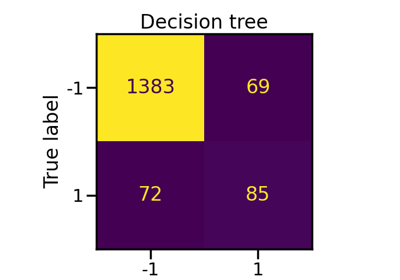# geometric_mean_score¶

`imblearn.metrics.``geometric_mean_score`(y_true, y_pred, *, labels=None, pos_label=1, average='multiclass', sample_weight=None, correction=0.0)[source]

Compute the geometric mean.

The geometric mean (G-mean) is the root of the product of class-wise sensitivity. This measure tries to maximize the accuracy on each of the classes while keeping these accuracies balanced. For binary classification G-mean is the squared root of the product of the sensitivity and specificity. For multi-class problems it is a higher root of the product of sensitivity for each class.

For compatibility with other imbalance performance measures, G-mean can be calculated for each class separately on a one-vs-rest basis when `average != 'multiclass'`.

The best value is 1 and the worst value is 0. Traditionally if at least one class is unrecognized by the classifier, G-mean resolves to zero. To alleviate this property, for highly multi-class the sensitivity of unrecognized classes can be “corrected” to be a user specified value (instead of zero). This option works only if `average == 'multiclass'`.

Read more in the User Guide.

Parameters
y_truendarray of shape (n_samples,)

Ground truth (correct) target values.

y_predndarray of shape (n_samples,)

Estimated targets as returned by a classifier.

labelslist, default=None

The set of labels to include when `average != 'binary'`, and their order if `average is None`. Labels present in the data can be excluded, for example to calculate a multiclass average ignoring a majority negative class, while labels not present in the data will result in 0 components in a macro average.

pos_labelstr or int, default=1

The class to report if `average='binary'` and the data is binary. If the data are multiclass, this will be ignored; setting `labels=[pos_label]` and `average != 'binary'` will report scores for that label only.

averagestr or None, default=’multiclass’

If `None`, the scores for each class are returned. Otherwise, this determines the type of averaging performed on the data:

`'binary'`:

Only report results for the class specified by `pos_label`. This is applicable only if targets (`y_{true,pred}`) are binary.

`'micro'`:

Calculate metrics globally by counting the total true positives, false negatives and false positives.

`'macro'`:

Calculate metrics for each label, and find their unweighted mean. This does not take label imbalance into account.

`'weighted'`:

Calculate metrics for each label, and find their average, weighted by support (the number of true instances for each label). This alters ‘macro’ to account for label imbalance; it can result in an F-score that is not between precision and recall.

`'samples'`:

Calculate metrics for each instance, and find their average (only meaningful for multilabel classification where this differs from `accuracy_score`).

sample_weightndarray of shape (n_samples,), default=None

Sample weights.

correction: float, default=0.0

Substitutes sensitivity of unrecognized classes from zero to a given value.

Returns
geometric_meanfloat

Notes

References

1

Kubat, M. and Matwin, S. “Addressing the curse of imbalanced training sets: one-sided selection” ICML (1997)

2

Barandela, R., Sánchez, J. S., Garcıa, V., & Rangel, E. “Strategies for learning in class imbalance problems”, Pattern Recognition, 36(3), (2003), pp 849-851.

Examples

```>>> from imblearn.metrics import geometric_mean_score
>>> y_true = [0, 1, 2, 0, 1, 2]
>>> y_pred = [0, 2, 1, 0, 0, 1]
>>> geometric_mean_score(y_true, y_pred)
0.0
>>> geometric_mean_score(y_true, y_pred, correction=0.001)
0.010000000000000004
>>> geometric_mean_score(y_true, y_pred, average='macro')
0.47140452079103168
>>> geometric_mean_score(y_true, y_pred, average='micro')
0.47140452079103168
>>> geometric_mean_score(y_true, y_pred, average='weighted')
0.47140452079103168
>>> geometric_mean_score(y_true, y_pred, average=None)
array([ 0.8660254,  0.       ,  0.       ])
```

## Examples using `imblearn.metrics.geometric_mean_score`¶